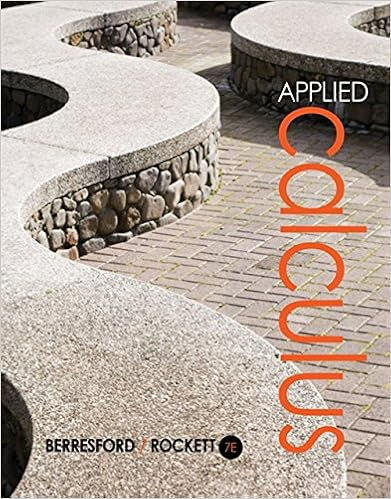# RIEMANN SUM AND DEFINITE INTEGRALS A Partition with...

• Notes
• 2

This preview shows page 1 - 2 out of 2 pages.

##### We have textbook solutions for you!
The document you are viewing contains questions related to this textbook.The document you are viewing contains questions related to this textbook.
Chapter 5 / Exercise 43
Applied Calculus
Berresford/RockettExpert Verified
4.3 RIEMANN SUM AND DEFINITE INTEGRALS A Partition with subintervals of n equal lengths Example1: Find the area bounded by the graph of f(x) = x 1/2 and the x-axis for x on [0, 1]. Sketch the graph: A) List right end-points in form of i. B) List the heights in form of i. C) Write the widths in form of i. D) Write the area using Sigma Notation. Find the actual area by finding limit of the sum as n approach infinity. DEFINITION OF THE RIEMANN SUM: Let f be defined on a closed interval [a, b], and let f be defined on a closed interval [a, b], and let be a partition of [a, b] given: a = x o < x 1 <x 2 …<x n-1 <x n =b where x i is the length of the i th subinterval. If c i is any point in the i th subinterval, then the sum , x i-1 <c i <x Is called the Riemann Sum of f for the partition . DEFINITE INTEGRALS If f is defined on the closed interval [a, b] and the limit: exists, then f is integrable on [a, b] and the limit is denoted: = The limit is called the definite integral of f from a to b. The number a is the lower limit of the i
##### We have textbook solutions for you!
The document you are viewing contains questions related to this textbook.The document you are viewing contains questions related to this textbook.
Chapter 5 / Exercise 43
Applied Calculus
Berresford/RockettExpert Verified
•••